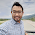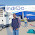# 10 bit A/D converters, the quantization error is given by (in Percent)-HAL 2011

B) 2

C) 0.1

D) 0.2

Note: Post your answers with Option name and reason of your answer so that others can able to understand and if you want to receive answers from other candidates click notify me below the comment form.

1..1%...............since quantiztion error is given by (1/no of step size).
total no of step size -2^n where n is the no of bit...
2^10=1024
q=(1/1024)
ie .1%

1.As per my knowledge Quantization error is given as + - (Step Size)/2
Step size= Vpp/2^10 = Vpp/1024
Qe=Vpp/2048

Percentage Qe= 1/2048 ~ 0.05%

Where I am making mistake?

2.Step Size is Vmax-Vmin i.e., 2v i.e,2v/2048

2.i m agree with above answer.